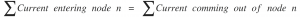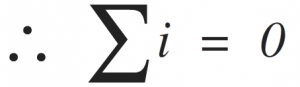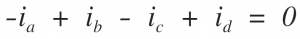Home > dccircuits > kirchhoffs current law

# Kirchhoff's Current Law

Kirchhoff's current law (KCL) is also called as point law. It states that the sum of currents directed into any node in a circuit is equal to the sum of the currents coming out of the same node.

Mathematically this can be expressed as :This can be stated in a slightly different way as :

The total sum of all the branch currents in a parallel circuit must be exactly equal to the source current OR the algebraic sum of all currents entering or leaving a node must be equal to zero.The first law is Kirchhoﬀ's current law(KCL), which states that the algebraic sum of currents entering any node is zero.Let us consider the node shown in Figure. The sum of the currents entering the node is,Note that we have since the current is leaving the node. If we multiply the foregoing equation by -1, we obtain the expression,which simply states that the algebraic sum of currents leaving a node is zero. Alternately, we can write the equation as,which states that the sum of currents entering a node is equal to the sum of currents leaving the node. If the sum of the currents entering a node were not equal to zero, then the charge would be accumulating at a node. However, a node is a perfect conductor and cannot accumulate or store charge. Thus, the sum of currents entering node is zero.